# A pulley and wedge system

• Differentiate it
The normal force is a force that acts perpendicular to the direction of motion. In this case, it is acting down the slope and up the slope on the mass M. It's... confusing.

#### Differentiate it

Homework Statement
There is no friction, and the pulley is massless. What is the acceleration of mass M?
Relevant Equations
F = ma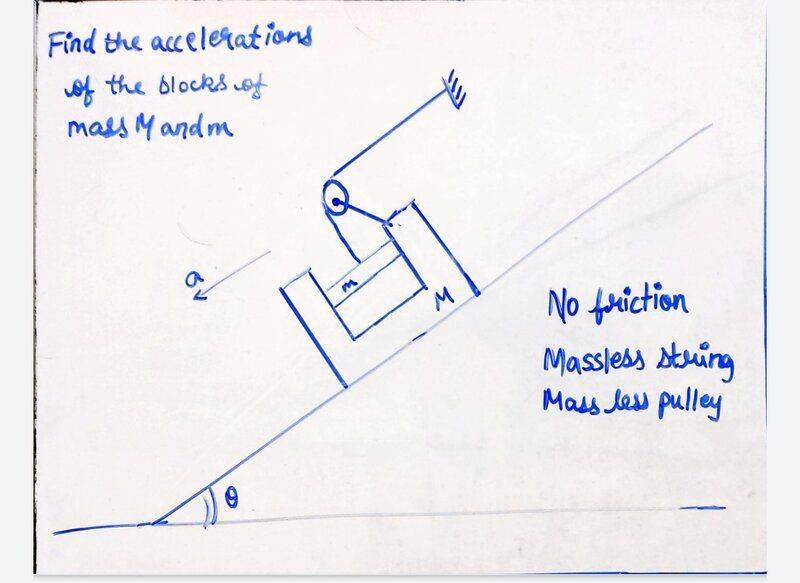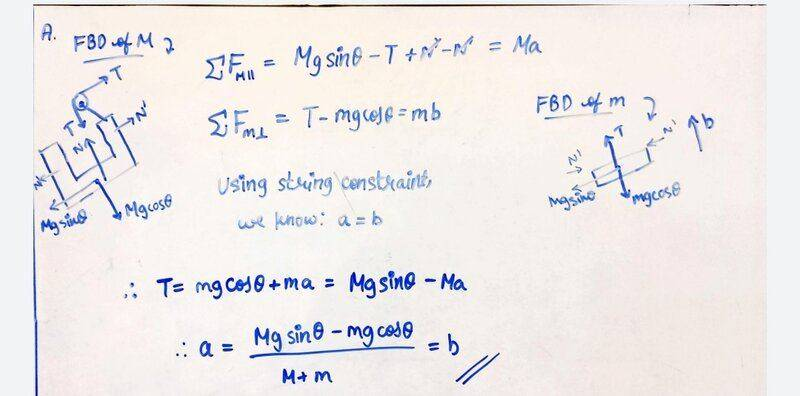Is my answer correct? And if not, why?

It does not look correct. When ##\theta = 0## (no incline) the acceleration of mass ##M## should be zero. Your expression predicts that it should be $$a=-\frac{m}{M+m}g.$$

kuruman said:
It does not look correct. When ##\theta = 0## (no incline) the acceleration of mass ##M## should be zero. Your expression predicts that it should be $$a=-\frac{m}{M+m}g.$$
So where did I go wrong?

Differentiate it said:
So where did I go wrong?
That I cannot tell you until I understand the setup shown in your drawing, specifically the disembodied piece of wall (upper right) to which the end of the string is attached. You show it just hanging in mid air which doesn't make much sense.

Differentiate it said:
So where did I go wrong?
kuruman said:
That I cannot tell you until I understand the setup shown in your drawing, specifically the disembodied piece of wall (upper right) to which the end of the string is attached. You show it just hanging in mid air which doesn't make much sense.
It's just a fixed wall perpendicular to the incline. Also, would this be the correct answer? I don't really think so, but I'm just confirming

#### Attachments

kuruman said:
It does not look correct. When ##\theta = 0## (no incline) the acceleration of mass ##M## should be zero. Your expression predicts that it should be $$a=-\frac{m}{M+m}g.$$
This is an incorrect conclusion. When the incline is zero there is a net horizontal force mg on the masses due to the tension in the string.

•berkeman
Orodruin said:
This is an incorrect conclusion. When the incline is zero there is a net horizontal force mg on the masses due to the tension in the string.
Hm, that makes sense. So could you confirm if my answer is correct. And if not, why?

Orodruin said:
This is an incorrect conclusion. When the incline is zero there is a net horizontal force mg on the masses due to the tension in the string.
Wait. If the mass M doesn't accelerate down the incline, the smaller mass m doesn't accelerate either l, so the tension is zero...

I've arrived at the second solution you've posted (which doesn't show any work). Given my track record, I estimate it gives it about a 10% chance of being correct.

How did you get to the second solution?

The funny thing is I think you could arrive at it, even with what I believe to be a false assumption that the Normal forces acting on the upper and lower portion of the blocks (where they contact each other) are equal in magnitude, but if you subbed that result back into any EoM in the ##x## direction you should get some kind of a contradiction.

Last edited:
Orodruin said:
This is an incorrect conclusion. When the incline is zero there is a net horizontal force mg on the masses due to the tension in the string.
Yes, you're right. I wasn't sure about the wall.
Differentiate it said:
It's just a fixed wall perpendicular to the incline. Also, would this be the correct answer? I don't really think so, but I'm just confirming
What do ##a## and ##b## represent? Are they supposed to be the accelerations of ##m## and ##M##? Please don't show just the bottom line of whatever work you did. How can we figure out where you went wrong if we don't know how you got there?

That said, consider taking the two masses together plus the pulley as your system. That would be all that is contained within the box with dotted lines in the picture. The external forces acting on this system are (a) Gravity ##(m+M)g##; (b) Normal force ##N##; (c) tension ##T##.

In the direction parallel to the incline you have
##T-(M+m)g\sin\!\theta=-(M+m)a.##

In the direction perpendicular to the incline you have
##N-(M+m)g\cos\!\theta=0.##

My point is that if you draw separate FBDs for ##M## and ##m##, the pairs of equations that you get should give the above two when added because the net force on ##m## plus the net force on ##M## is the net force on ##M+m##.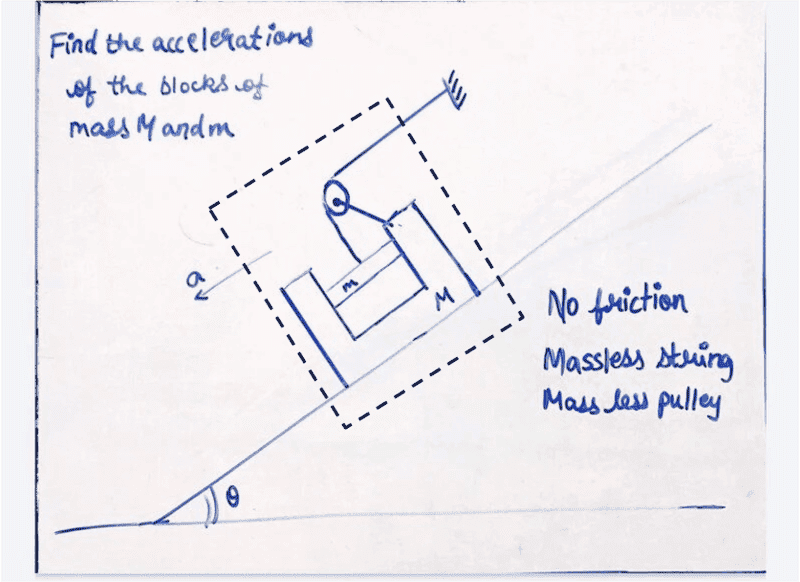Differentiate it said:
Wait. If the mass M doesn't accelerate down the incline, the smaller mass m doesn't accelerate either l, so the tension is zero...
In the case with no incline the mass M accelerates to the right because the mass m is being dragged down. By simple geometry, the acceleration of m is the same as that of M horizontally and of the same magnitude and pointing down vertically.

•Differentiate it
Also just to point out that this is one of the cases where Lagrange mechanics makes things so much simpler by basically just giving you the solution.

Differentiate it said:
Homework Statement:: There is no friction, and the pulley is massless. What is the acceleration of mass M?
Relevant Equations:: F = ma

View attachment 313426
Is my answer correct? And if not, why?
I don't think you handled the normal force ##N'## correctly.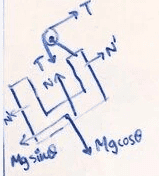You have ##N'## acting simultaneously down the slope and up the slope on ##M##. It's either one or the other, but not both. You can assume either one and then if you get a negative answer for ##N'## it means that ##N'## is acting in the opposite direction from your initial choice.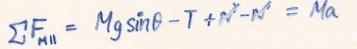, ##N'## doesn't cancel.

You'll need an additional equation. What about an equation for ##\sum F_{m, ||}## for the piston?

Differentiate it said:
Also, would this be the correct answer? I don't really think so, but I'm just confirming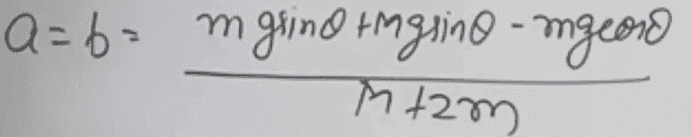This looks correct to me.

#### Attachments

Since the correct answer has been arrived at by OP, here is the Lagrange approach:

Distance of ##M## from top ##x##. The distance of ##m## from top along the incline is also ##x##. The perpendicular distance of ##m## from ramp is ##\ell + x##.
Potential energy is
$$V = -(m+M)g x \sin\theta + mg (\ell + x)\cos\theta.$$
Kinetic energy is
$$T = \frac{M}2 \dot x^2 + m\dot x^2.$$
Equations of motion directly follow
$$\frac{d}{dt}(\partial_{\dot x} T) =(M + 2m)\ddot x = -\partial_x V = (m+M)g\sin\theta + mg\cos\theta$$
or equivalently
$$a = \frac{ (m+M)g\sin\theta - mg\cos\theta}{M + 2m}$$

Last edited:
•erobz and TSny
TSny said:
I don't think you handled the normal force ##N'## correctly.
View attachment 313454

You have ##N'## acting simultaneously down the slope and up the slope on ##M##. It's either one or the other, but not both. You can assume either one and then if you get a negative answer for ##N'## it means that ##N'## is acting in the opposite direction from your initial choice.

So, in your equation View attachment 313455, ##N'## doesn't cancel.

You'll need an additional equation. What about an equation for ##\sum F_{m, ||}## for piston?
Here is where over idealization runs into an issue then. The OP solved it by assuming the normal forces between ##M## and ##m## have equal but opposite magnitude, which can get you to the solution. I assumed they were different, but none the less present...and get to that answer. You say it's one or the other(upper or lower)...and I assume that will lead to the answer.

Without friction between any surfaces, ##m## could have an interference fit ##M## and it would make no difference in the solution, but it sure will change what those normal forces are.

Last edited:
erobz said:
Here is where over idealization runs into an issue then. The OP solved it by assuming the normal forces between ##M## and ##m## have equal but opposite magnitude, which can get you to the solution. I assumed they were different, but none the less present...and get to that answer. You say it's one or the other(upper or lower)...and I assume that will lead to the answer.
If the piston of mass m exerts a force ##N'## on M, then M will exert an equal but opposite force on m. But, in @Differentiate it 's free body diagram for M, there are two ##N'## forces acting on just M. This is confirmed by his/her equation for ##\sum F_{M ||}## for M which includes both ##N'## and ##-N'##. Likewise for the free body diagram for the piston.

Orodruin said:
$$\frac{d}{dt}(\partial_{\dot x} T) =(M + 2m)\ddot x = -\partial_x V = (m+M)g\sin\theta + mg\cos\theta$$
A sign error for the ##\cos \theta## term?

TSny said:
If the piston of mass m exerts a force ##N'## on M, then M will exert an equal but opposite force on m. But, in @Differentiate it 's free body diagram for M, there are two ##N'## forces acting on just M. This is confirmed by his/her equation for ##\sum F_{M ||}## for M which includes both ##N'## and ##-N'##. Likewise for the free body diagram for the piston.
Yeah I see that..I think. But I'm not sure you are seeing what I'm saying.

I have for the piston ## ^+ \swarrow x ##:

$$N_u - N_l + mg \sin \theta = m a$$

And for the Sleeve:

$$N_l - N_u -T + Mg \sin \theta = M a$$

If the piston is in some interference fit without friction and accelerating this seems fine to me?

With summing forces in the ##y## (normal to the slope) direction for the piston and applying the cord constraint:

$$T = m a + mg \cos \theta$$

And I get the solution.

One could easily assume ## | N_u | = | N_l |## and also get the same solution. And it seems like given the interference fit circumstances without friction it's not an impossibility, ## | N_u |## could in fact equal ##| N_l |##? am I missing something?

Last edited:
Another approach is conservation of energy. Let ##x## denote the displacement of M down along the slope from its initial position. Let the system start from rest and take the initial gravitational potential energy of the system to be zero. So, at any time after release, the total energy E = KE + PE is zero. $$\frac{1}{2}M\dot x^2 + \frac{1}{2}m(\sqrt 2 \dot x)^2 - (M+m)g \sin \theta \, x + m g \cos \theta \, x = 0$$ Take the time derivative of this equation and cancel the common factor of ##\dot x## in the result. There's quite a bit of similarity of this calculation to the Lagrangian calculation of @Orodruin 's post #15.

erobz said:
Yeah I see that..I think.

But I have for the piston ## ^+ \swarrow x ##:

$$N_u - N_l + mg \sin \theta = m a$$
I guess there could be both an ##N_u## and an ##N_l## force acting on ##m##. But, you could let ##N' \equiv N_u - N_l## and work with just ##N'##. Thus $$N' + mg \sin \theta = ma$$

erobz said:
And for the Sleeve:

$$N_u - N_l -T + Mg \sin \theta = M a$$
Wouldn't the signs of ##N_u## and ##N_l## be changed here? Using ##N' = N_u - N_l##, this equation would then be $$-N' -T + Mg \sin \theta = M a$$

•erobz
TSny said:
Wouldn't the signs of ##N_u## and ##N_l## be changed here? Using ##N' = N_u - N_l##, this equation would then be $$-N' -T + Mg \sin \theta = M a$$
Yep...transcription mistake. Fixed

•TSny
TSny said:
There's quite a bit of similarity of this calculation to the Lagrangian calculation of @Orodruin 's post #15.
That would be because the conservation of energy is a first integral of the Lagrangian equations of motion when the Lagrangian is time independent.

•TSny
TSny said:
A sign error for the ##\cos \theta## term?
Yes, fixed now. Copy paste error.

TSny said:
I guess there could be both an ##N_u## and an ##N_l## force acting on ##m##. But, you could let ##N' \equiv N_u - N_l## and work with just ##N'##. Thus $$N' + mg \sin \theta = ma$$

Wouldn't the signs of ##N_u## and ##N_l## be changed here? Using ##N' = N_u - N_l##, this equation would then be $$-N' -T + Mg \sin \theta = M a$$
How did you get this equation? Also , I think N' + mgsinθ = ma is incorrect.
I think it is T - mgcosθ = mb. I'm sorry for not mentioning, but a is the acceleration of M with respect to the ground and b is the acceleration of m with respect to M.

Last edited:
Differentiate it said:
How did you get this equation? Also , I think N' + mgsinθ = ma is incorrect.
I think it is T - mgcosθ = mb. I'm sorry for not mentioning, but a is the acceleration of M with respect to the ground and b is the acceleration of m with respect to M.
Please look into my solution again and tell me where I went wrong. The second image is the correct answer. If I forgot to mention,
•a is the acceleration of M with respect to the ground
•b is the acceleration of m with respect to M.
•I also know that a = b
And that's how I got my answer. Is there something wrong in my FBD's? Or is it something else?

#### Attachments

Differentiate it said:
Please look into my solution again and tell me where I went wrong. The second image is the correct answer. If I forgot to mention,
•a is the acceleration of M with respect to the ground
•b is the acceleration of m with respect to M.
•I also know that a = b
And that's how I got my answer. Is there something wrong in my FBD's? Or i
You shouldn't assume (as you have) the normal forces are equal in magnitude. You don't know that. Assuming an interference fit, it would be a very specific acceleration that would have ## |N_l| = |N_u|##. Technically we don't even know if there are two normal forces acting between the masses, in practice it would depend on the fit between the bodies.

## N_l ## is the normal force acting on the lower sided of the masses and ##N_u## the upper side.

If the fit is loose, then at the very least you must have 1 normal force ( whether or not the hanging mass is sliding up the upper of lower side. Your first attempt has no net normal forces whatsoever.

erobz said:
You shouldn't assume (as you have) the normal forces are equal in magnitude. You don't know that. Assuming an interference fit, it would be a very specific acceleration that would have ## |N_l| = |N_u|##. Technically we don't even know if there are two normal forces acting between the masses, it practices it would depend on the fit.

## N_l ## is the normal force acting on the lower sided of the masses and ##N_u## the upper side.

If the fit is loose, then at the very least you must have 1 normal force ( whether or not the hanging mass is sliding up the upper of lower side. Your first attempt has no net normal forces whatsoever.
It fits in perfectly, so, in that case, the normal forces are the same, right?

Differentiate it said:
It fits in perfectly, so, in that case, the normal forces are the same, right?
No, gravity would pull it to the lower side ( if the system was static ), and it would have a single Normal.

If it were a squeezed fit ( force fit ) it would have two Normals. However, they would not be equal in general for two reasons in this problem...gravity, and acceleration.

erobz said:
no, gravity would pull it to the lower side.

If it were a squeezed fit ( force fit ) it would have two Normals. However, they would not be equal in general for two reasons in this problem...gravity, and acceleration.
I believe in my equation
Mgsinθ - T +N' - N'
I have taken gravity into account

Differentiate it said:
I believe in my equation
Mgsinθ - T +N' - N'
I have taken gravity into account
No, you have pretended the bar is:

1) Horizontal in the sleeve
2) Squeeze fit into the sleeve so that it is contacting both sides.

Rotate that assembly by 90 degrees...do those Normal forces change?

Now accelerate that assembly like an elevator...did they change again?

erobz said:
No, you have pretended the bar is:

1) Horizontal in the sleeve
2) Squeeze fit into the sleeve so that it is contacting both sides.

Rotate that assembly by 90 degrees...do those Normal forces change?

Now accelerate that assembly like an elevator...did they change again?
Did I not mention that? Yeah, it's contacting both sides and is horizontal in the sleeve

Differentiate it said:
Did I not mention that? Yeah, it's contacting both sides and is horizontal in the sleeve
But its not horizontal in this problem. It is rotated from horizontal by the angle ##\theta##, and it is accelerating down the incline at ##a##.

erobz said:
But its not horizontal in this problem. It is rotated from horizontal by the angle ##\theta##, and it is accelerating down the incline at ##a##.
Well yes, but how does that affect the normal forces exerted by it on M?

Differentiate it said:
Well yes, but how does that affect the normal forces exerted by it on M?
You won't need to worry about that to solve the problem, but you can figure that out after if you like once you have the proper set of equations.